# Mean Function in MATLAB

• Last Updated : 29 Jun, 2021

Mean or average is the average of a sequence of numbers. In MATLAB, mean (A) returns the mean of the components of A along the first array dimension whose size doesn’t equal to 1. Suppose that A is a vector, then mean(A) returns the mean of the components. Now, if A is a Matrix form, then mean(A) returns a row vector containing the mean of every column.

MeanExample:

Mean of sequence x = [1,2,3,4,5]  = Sum of numbers/Count of numbers

= 15/5

= 3

Different syntax of the mean() method is:

• M = mean(A)
• M = mean(A,’all’)
• M = mean(A,dim)
• M = mean(A,vecdim)

### M = mean(A)

• It returns the mean of sequence A.
• If A is a vector, then it returns the mean of all elements in the vector
• If A is a matrix, then it returns a vector where each element is the mean of each column in A.

Example:

## Matlab

 % Input vectorA = [1 2 3 4 5];disp("Vector :");disp(A); % Find mean of vectorx = mean(A);disp("Mean :");disp(x);

Output: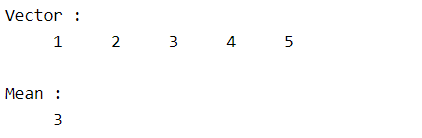Example:

## Matlab

 % Input matrixA = [1 1 2; 2 3 2; 0 1 2; 1 5 7];disp("Matrix :");disp(A); % Find mean of matrixx = mean(A);disp("Mean :");disp(x);

Output :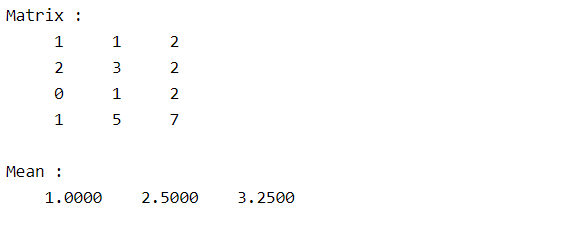### M = mean(A, ‘all’)

It returns the mean of all the elements in A either it can be vector or matrix.

Example:

## Matlab

 % Input matrixA = [1 1 2; 2 3 2; 0 1 2; 1 5 7];disp("Matrix :");disp(A); % Find mean of whole matrixx = mean(A,'all');disp("Mean :");disp(x);

Output :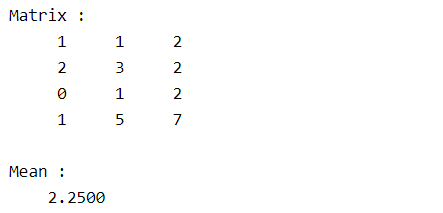### M = mean(A,dim)

• It returns the mean of matrix A along each of the given dim.
• If dim = 1, then it returns a vector where the mean of each column is included.
• If dim = 2, then it returns a vector where the mean of each row is included.

Example:

## Matlab

 % Input matrixA = [1 1 2; 2 3 2; 0 1 2; 1 5 7];disp("Matrix :");disp(A); % Find mean of each row in matrixx = mean(A,2);disp("Mean :");disp(x);

Output :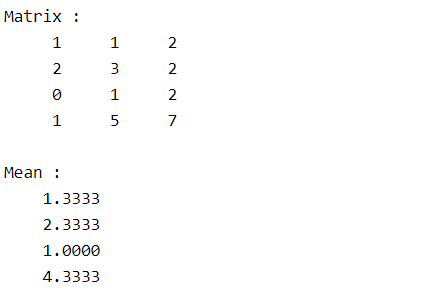### M = mean(A,vecdim)

• It returns the mean of A based on the specified dimensions vecdim in A.
• If A is a 2-by-2-by-3 array, then mean(A,[1 2]) calculates the mean of each page of size 2-by-2 as it’s considered as a single entity. So it returns the vector of size 3 as the mean of each page.

Example:

## Matlab

 % Creating a 2-by-3-by-3 arrayA(:,:,1) = [12 2; -1 1];A(:,:,2) = [3 13; -2 10];A(:,:,3) = [4 7 ; 3 -3];disp("Array :");disp(A); % Calculate mean of each pageM1 = mean(A,[1 2]);disp("Mean of each page :");disp(M1);

Output: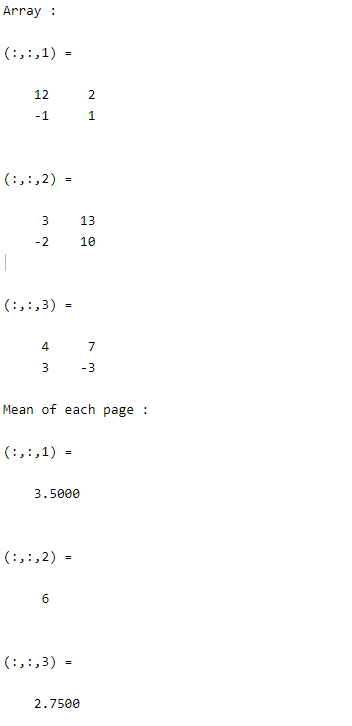My Personal Notes arrow_drop_up